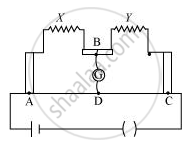Department of Pre-University Education, KarnatakaPUC Karnataka Science Class 12
Advertisement Remove all ads

# The Figure Shows Experimental Set up of a Meter Bridge. When the Two Unknown Resistances X and Y Are Inserted, the Null Point D is Obtained 40 Cm from the End A. When a Resistance of 10 ω Is Connected - Physics

Advertisement Remove all ads
Advertisement Remove all ads
Advertisement Remove all adsThe figure shows experimental set up of a meter bridge. When the two unknown resistances X and Y are inserted, the null point D is obtained 40 cm from the end A. When a resistance of 10 Ω is connected in series with X, the null point shifts by 10 cm. Find the position of the null point when the 10 Ω resistance is instead connected in series with resistance ‘Y’. Determine the values of the resistances X and Y.

Advertisement Remove all ads

#### Solution

For a metre bridge:

X/Y = l_1/(100 - l_1)… (1)

Where, it is given that l1 = 40 cm

X/Y = 40/(100 -40)  = 2/3… (2)

When 10 Ω resistance is added in series to X, null point shifts by 10 cm.

(X+10)/Y  = (40+10)/(100 - (40 +10))

X +10 = 50/50

(X+10)/Y = 1 or X+10 = Y   ..... (3)

Substituting the value of X from equation (2), we obtain

2/3y+10 = Y

10 = Y -2/3 Y

or

Y/3 =10

Y =30 Omega

Substituting the value of Y in equation (3), we obtain

X + 10 = 30

= 20 Ω

Position of the null point when 10 Ω resistance is put in series with Y,

20/(30+10) = l_i/(100 - l_i)

2000 - 20l_1 = 40l_1

60l_1 = 2000

l_1 = 2000/60

l_1 = 33.3 cm

Concept: Metre Bridge
Is there an error in this question or solution?

#### Video TutorialsVIEW ALL 

Advertisement Remove all ads
Share
Notifications

View all notifications

Forgot password?